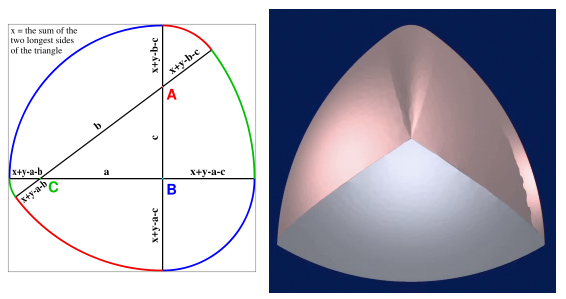# Are there smooth bodies of constant width?

The standard Reuleaux triangle is not smooth, but the three points of tangential discontinuity can be smoothed as in the figure below (left), from the Wikipedia article. However, it is unclear (to me) from this diagram whether the curve is $C^2$ or $C^\infty$.

Meissner’s tetrahedron is a 3D body of constant width, but it is not smooth, as is evident in the right figure below.My question is:

Are there $C^\infty$ constant-width bodies in $\mathbb{R}^d$ (other than the spheres)?

The image of Meissner’s tetrahedron above is taken from the impressive work of Thomas Lachand–Robert and Edouard Oudet, "Bodies of constant width in arbitrary dimension" (Math. Nachr. 280, No. 7, 740-750 (2007); pre-publication PDF here).

I suspect the answer to my question is known, in which case a reference would suffice. Thanks!

Addendum. Thanks to the knowledgeable (and rapid!) answers by Gerry, Anton, and Andrey, my question is completely answered—I am grateful!!

• I trust you mean, other than the sphere. – Gerry Myerson Feb 3 '11 at 22:40
• @Gerry: Yes, that's what I meant, thanks; updated the question. – Joseph O'Rourke Feb 3 '11 at 22:48
• You ask whether the curve on the diagram is smooth. It isn't: it's made from six circle arcs. – Zsbán Ambrus Feb 4 '11 at 10:25
• Thanks, Zsbán. So I guess just $C^1$? – Joseph O'Rourke Feb 4 '11 at 10:45

Fillmore showed that there are sets of constant width in $\mathbb R^d$ with analytic boundaries which have a trivial symmetry group (so these are very different from spheres; see "Symmetries of surfaces of constant width", J. Differential Geom., Vol 3, (1969), pp. 103-110).

Moreover, the set of bodies of constant width with analytic boundaries is dense in the space of all convex bodies of constant width in $\mathbb R^d$ with respect to the Hausdorff metric (see e.g. "Smooth approximation of convex bodies" by Schneider).

• In the second paragraph, a "of constant width" is missing; it is implicit, but it disturbed me for a second. – Benoît Kloeckner Feb 11 '11 at 12:11
• For an ignoramus like me, these results are quite impressive and highly counter-intuitive. – Olivier Dec 11 '11 at 14:19
• I took a look at the Fillmore paper, and just before his Corollary to Theorem 2 -- which reads "Corollary. There exists an analytic hypersurface of constant width in E^n having the same group of symmetries as a regular n-simplex." -- he writes "If we imitate the construction of a Reuleux triangle . . .. Thus:" This seems to imply that he is assuming that [the intersection of four balls in 3-space, centered at the vertices of a regular tetrahedron and each with radius = the side-length of the tetrahedron] is a body of constant width. But this is known to be false. – Daniel Asimov Jan 11 '13 at 4:50

Take any odd $C^\infty$-function $f$ on the sphere. Consider the convex set $$R_\epsilon=\{\,x\in\mathbb R^n\mid\langle x,u\rangle\le 1+\epsilon{\cdot}f(u)\ \ \text{for any}\ \ u\in\mathbb{S}^{n-1}\,\}.$$ Note that for all sufficiently small $\epsilon>0$, $R_\epsilon$ is a smooth body of constant width.

Jay P Fillmore, Symmetries of surfaces of constant width, J Differential Geometry 5 (1969) 103-110, says: the curve $$x_1=h\cos\theta-{dh\over d\theta}\sin\theta,\qquad x_2=h\sin\theta+{dh\over d\theta}\cos\theta$$ where $h=a+b\cos3\theta$, $0\lt8b\lt a$, is analytic and of constant width. If we rotate this curve in Euclidean space ${\bf E}^n$ about an $(n-2)$-dimensional axis perpendicular to the line $\theta=0$, we obtain an analytic surface, not a sphere, of constant width in ${\bf E}^n$.

The paper may be available at http://www.intlpress.com/JDG/archive/1969/3-1&2-103.pdf

Take any odd smooth function h on the unit (d-1)-sphere and take a constant r>0 large enough to ensure that h+r is the support function of a convex body K

(the condition for h+r to be the support function of a smooth convex body whose boundary has positive Gaussian curvature is that the eigenvalues of Hess(h)+(h+r).Id be positive).

This convex body K is of constant width 2r.

Moreover, any smooth convex body with constant width 2r whose boundary has positive Gaussian curvature can be constructed in this way :

If S is a closed convex hypersurface of constant width 2r, then S is the sum of a sphere of radius r with a "projective hedgehog" H whose support function h is the odd part of the support function of S (and which can be regarded as the locus of the middles of S's diameters)." ;

See for instance:

Y. Martinez-Maure, Arch. Math., Vol. 67, 156-163 (1996), page 157.

This relevant paper was just released:

Howard Resnikoff. "On Curves and Surfaces of Constant Width." Apr. 2015. (arXiv abstract.)

"...we provide a Fourier series-based construction that produces arbitrarily many new surfaces of constant width."Michael Kallay characterized the set of all planar sets with a given width functions: See M. Kallay, Reconstruction of a plane convex body from the curvature of its boundary. Israel J. Math. 17 (1974), 149–161. and M. Kallay, The extreme bodies in the set of plane convex bodies with a given width function. Israel J. Math. 22 (1975), no. 3-4, 203–207.